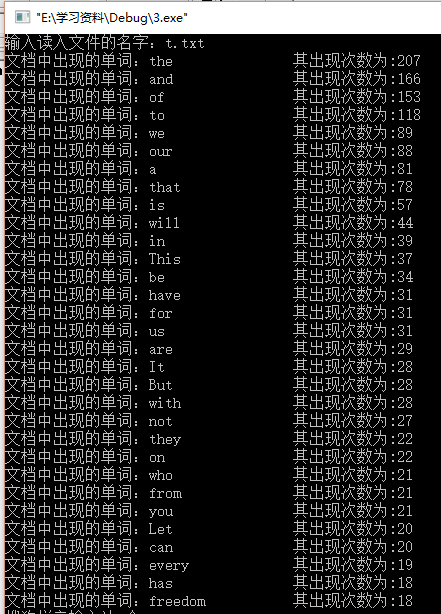# c语言实现词频统计的简单实例

更新时间：2016年09月06日 14:44:04   投稿：jingxian

1.设计一个词频统计软件，统计给定英文文章的单词频率。

2.文章中包含的标点不计入统计。

3.将统计结果以从大到小的排序方式输出。

1.因为是跨专业0.0・・・并不会c++和java，只能用仅学过的C语言进行编写，还是挺费劲的。

2.定义一个包含单词和频率两个成员的结构体来统计词频（进行了动态分配内存，可以处理较大文本）。

3.使用fopen函数读取指定的文档。

4.使用fgetc函数获取字符，再根据取得的字符是否是字母进行不同的处理。

5.采用快速排序法对统计结果进行排序。

5.将整个统计结果循环输出。

```struct fre_word
{
int num;
char a;
}; ```

```struct fre_word *w;
w=(struct fre_word *)malloc(100*p*sizeof(struct fre_word));//给结构体分配初始内存```

```printf("输入读入文件的名字：");
scanf("%s", filename);                   //输入需要统计词频的文件名
if((fp=fopen(filename, "r"))==NULL)
{
printf("无法打开文件\n");
exit(0);
}```

```/****************将单词出现次数设置为1****************************/
for(i=0;i<100;i++)
{
(w+i)->num=1;
}
/****************单词匹配****************************************/
i=0;
while(!feof(fp))//文件尚未读取完毕
{
ch=fgetc(fp);
(w+i)->a[j]='\0';
if(ch>=65&&ch<=90||ch>=97&&ch<=122)              //ch若为字母则存入
{
(w+i)->a[j]=ch;
j++;
flag=0;                         //设标志位判断是否存在连续标点或者空格
}
else if(!(ch>=65&&ch<=90||ch>=97&&ch<=122)&&flag==0)    //ch若不是字母且上一个字符为字母
{
i++;
j=0;
flag=1;
for(m=0;m<i-1;m++)                  //匹配单词，若已存在则num+1
{
if(stricmp((w+m)->a,(w+i-1)->a)==0)
{
(w+m)->num++;
i--;
}
}
}
/****************动态分配内存****************************************/
if(i==(p*100))                           //用i判断当前内存已满
{
p++;
w=(struct fre_word*)realloc(w,100*p*(sizeof(struct fre_word)));
for(n=i;n<=100*p;n++)                      //给新分配内存的结构体赋初值
(w+n)->num=1;

}
}```

```void quick(struct fre_word *f,int i,int j)
{
int m,n,temp,k;
char b;
m=i;
n=j;
k=f[(i+j)/2].num;              //选取的参照
do
{
while(f[m].num>k&&m<j) m++;       // 从左到右找比k小的元素
while(f[n].num<k&&n>i) n--;       // 从右到左找比k大的元素
if(m<=n)
{                    //若找到且满足条件，则交换
temp=f[m].num;
strcpy(b,f[m].a);
f[m].num=f[n].num;
strcpy(f[m].a,f[n].a);
f[n].num=temp;
strcpy(f[n].a,b);
m++;
n--;
}
}
while(m<=n);
if(m<j) quick(f,m,j);           //运用递归
if(n>i) quick(f,i,n);
}```

```for(n=0;n<=i;n++)
{
printf("文档中出现的单词：");
printf("%-18s",(w+n)->a);
printf("其出现次数为:");
printf("%d\n",(w+n)->num);
}```